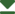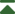p11a m Monoclinic/Oblique No. 5 p11a Patterson symmetry p112/m CELL CHOICE 1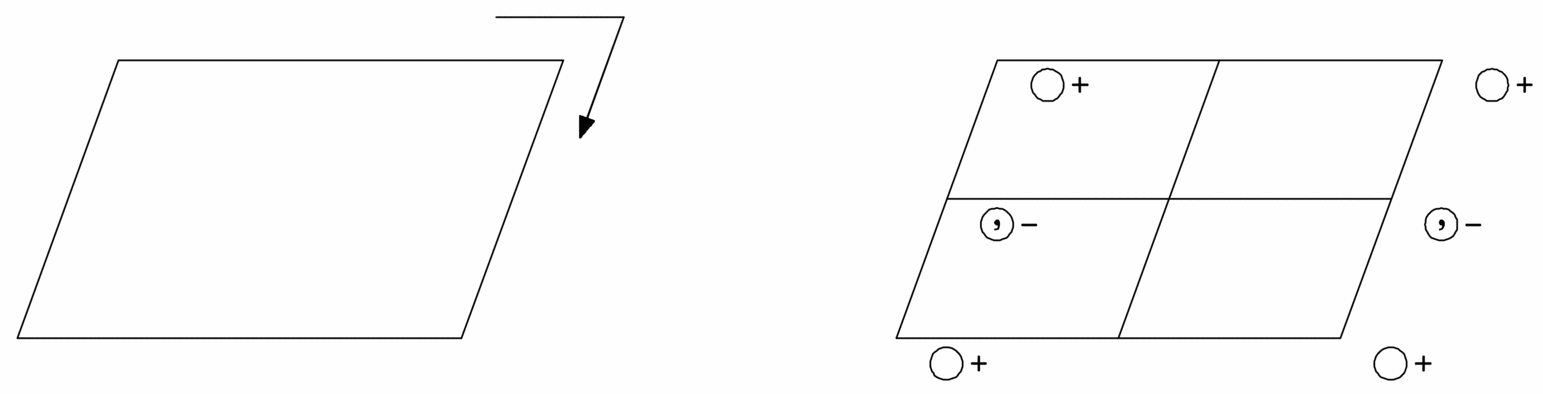Origin on glide plane a

 Asymmetric unit 0 ≤ x ≤ 1; 0 ≤ y ≤ 1; 0 ≤ z

Symmetry operations

 (1)  1 (2)  a   x, y, 0

Generators selected (1); t(1, 0, 0); t(0, 1, 0); (2)

Positions

Multiplicity, Wyckoff letter,
Site symmetry
Coordinates Reflection conditions

General:
 2 a 1
 (1) x, y, z (2) x + 1/2, y, -z
hk: h = 2n
h0: h = 2n

Symmetry of special projections

 Along    p1 a' = 1/2a   b' = b    Origin at 0, 0, z Along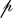11m a' = bp    Origin at x, 0, 0 Along11g a' = ap    Origin at 0, y, 0

Maximal non-isotypic subgroups

 I  p1 (1) 1
 IIa none
 IIb none

Maximal isotypic subgroups of lowest index

 IIc  p11a (b' = 2b or a' = a + 2b, b' = 2b) (5)

Minimal non-isotypic supergroups

 I  p112/a (7);  pm21b (28);  pb2b (30);  pm2a (31);  pm21n (32);  pb21a (33);  pb2n (34);  cm2e (36)
 II  p11m (a' = 1/2a) (4)

DIFFERENT CELL CHOICES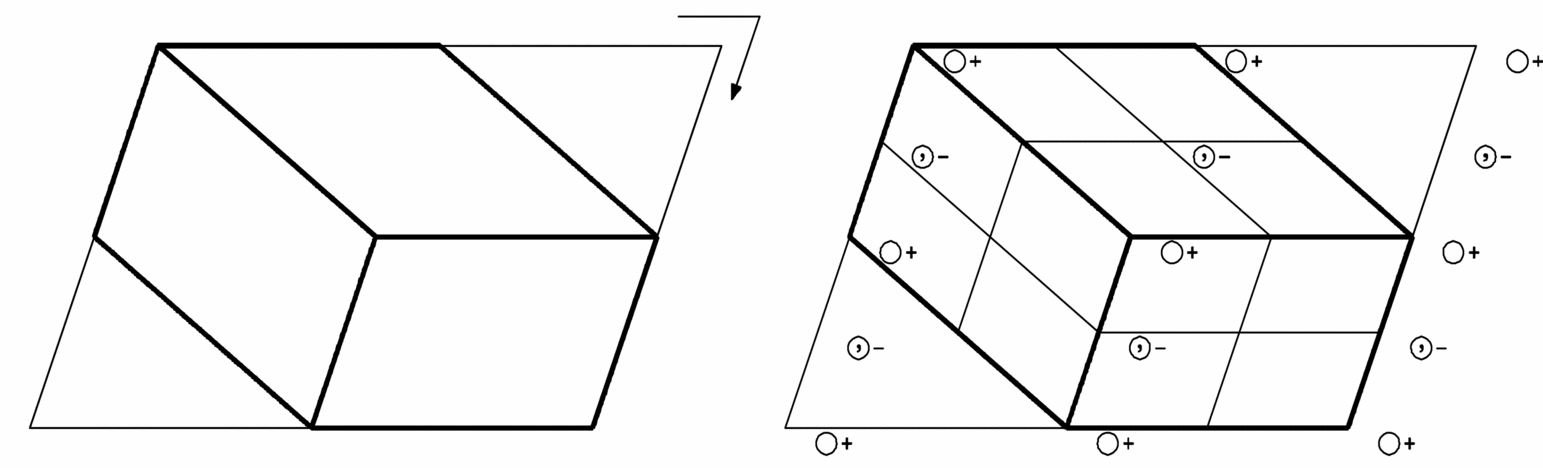p11a

CELL CHOICE 1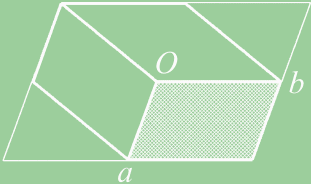Origin on glide plane a

 Asymmetric unit 0 ≤ x ≤ 1; 0 ≤ y ≤ 1; 0 ≤ z

Generators selected (1); t(1, 0, 0); t(0, 1, 0); (2)

Positions

Multiplicity, Wyckoff letter,
Site symmetry
Coordinates Reflection conditions

General:
 2 a 1
 (1) x, y, z (2) x + 1/2, y, -z
hk: h = 2n
h0: h = 2n

p11n

CELL CHOICE 2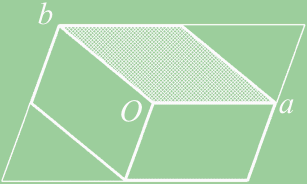Origin on glide plane n

 Asymmetric unit 0 ≤ x ≤ 1; 0 ≤ y ≤ 1; 0 ≤ z

Generators selected (1); t(1, 0, 0); t(0, 1, 0); (2)

Positions

Multiplicity, Wyckoff letter,
Site symmetry
Coordinates Reflection conditions

General:
 2 a 1
 (1) x, y, z (2) x + 1/2, y + 1/2, -z
hk: h + k = 2n
h0: h = 2n
0k: k = 2n

p11b

CELL CHOICE 3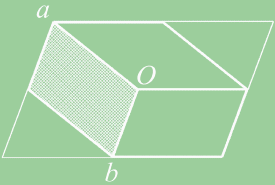Origin on glide plane b

 Asymmetric unit 0 ≤ x ≤ 1; 0 ≤ y ≤ 1; 0 ≤ z

Generators selected (1); t(1, 0, 0); t(0, 1, 0); (2)

Positions

Multiplicity, Wyckoff letter,
Site symmetry
Coordinates Reflection conditions

General:
 2 a 1
 (1) x, y, z (2) x, y + 1/2, -z
hk: k = 2n
0k: k = 2n